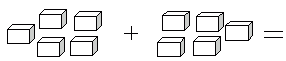Name: ___________________Date:___________________

 Email us to get an instant 20% discount on highly effective K-12 Math & English kwizNET Programs!

Grade 1 - Mathematics3.25 Computation Review Test

 Q 1: Which addition fact does not belong for the sum of 55 + 0 = 54 + 1 = 53 + 2 = 52 + 4 = 6 Q 2: Write 6 + 7 in doubles plus one form.6 + 6 + 1 = 135 + 5 + 3 = 137 + 4 + 2 = 138 + 2 + 3 = 13 Q 3: Which addition fact does not belong for the sum of 71 + 1 = 22 + 5 = 77 + 0 = 73 + 4 = 7 Q 4: Count the number of things and write the answer.Answer: Q 5: Using the Hundred Table, find the missing number in the figure.Answer: Q 6: Half of 8 is4816 Q 7: Double 4 you get428 Q 8: Sam ate 12 cookies on Monday and 6 on Tuesday. How many cookies did he eat in all?17192018 Question 9: This question is available to subscribers only! Question 10: This question is available to subscribers only!